Share

Books Shortlist
Your shortlist is empty

# Selina solutions for Class 10 Physics chapter 8 - Current Electricity

## Selina ICSE Concise Physics for Class 10

#### Selina Selina ICSE Concise Physics Class 10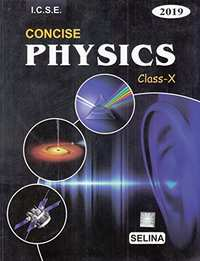## Chapter 8: Current Electricity

#### Chapter 8: Current Electricity solutions [Page 0]

Define the term current and state its S.I unit.

Define the term electric potential. State its S.I. unit.

How is the electric potential difference between the two points defined? State its S.I. unit.

Explain the statement ‘the potential difference between two points is 1 volt’.

Explain the analogy between the flow of charge (or current) in a conductor under a potential difference with the free fall of a body under gravity.

Define the term resistance. State its S.I. unit.

Name the particles which are responsible for the flow of current in a metal. Explain the flow of current in a metal on the basis of movement of the particles name by you.

How does the resistance of a wire depend on its radius? Explain your answer.

How does the resistance of a wire depend on its length? Give a reason for your answer with reason.

How does the resistance of a metallic wire depend on its temperature? Explain with reason.

Two wires one of copper and other of iron, are of the same length and same radius. Which will have more resistance? Give reason.

Name three factors on which resistance of a given wire depends and state how is it affected by the factors stated by you.

State Ohm’s law and draw a neat labelled circuit diagram containing a battery, a key, a voltmeter, an ammeter, a rheostat and an unknown resistance to verify it.

What is the necessary condition for a conductor to obey Ohm’s law?

draw a V-I graph for a conductor obeying Ohm’s law. (b) what does the slope of V–I graph  for a conductor represent?

Draw a I – V graph for a linear resistor. What does its slope represent?

What is an ohmic resistor? Give one example of an ohmic resistor. Draw a graph to show its current voltage relationship. How is the resistance of the resistor determined from this graph.

What are non-ohmic reistors? Give one example and draw a graph to show its current-voltage relationship.

Give two difference between an ohmic and non-ohmic resistor.

Figure , below shows the I-V characteristic curves for four resistors. Identify the ohmic and non-ohmic resistors. Give a reason for your answer.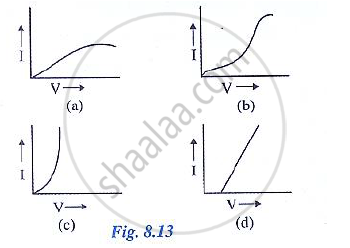Draw a V-I graph for a conductor at two different temperature. What conclusion do you draw from your graph for the variation of resistance of conductor with temperature?

Define the term resistivity and state its S.I unit.

Write an expression connecting the resistance and resistivity. State the meaning of symbols used.

State the order of resistivity of (i) a metal, (ii) a semiconductor and (iii) an insulator.

Name a substance of which the resistance remains almost unchanged by the increase in  temperature.

Name the material used for making the connection wires. Give a reason for your answer. Why
should a connection wire be thick?

Name a material which is used for making the standard resistor. Give a reason for your answer.

Name the material used for making a fuse wire. Give a reason.

Name the material used for  filament of an electric bulb .

Name the material used for heating element of a room heater.

What is a superconductor? Give one example of it.

A substance has zero resistance below 1 k. what is such a substance called?

#### Chapter 8: Current Electricity solutions [Page 0]

Which of the following is an ohmic resistance?
(a) diode valve

(b) junction diode

(c) filament of a bulb

(d) nichrome

For which of the following substance, resistance decreases with increase in temperature?
(a) copper

(b) mercury

(c) carbon

(d) platinum

#### Chapter 8: Current Electricity solutions [Page 0]

In a conductor 6.25 × 10^16 electrons flow from its end A to B in 2 s. Find the current flowing
through the conductor (e = 1.6 × 10^19 C)

A current of 1.6 mA flows through a conductor. If charge on an electron is – 1.6 × 10^-19 coulomb,
find the number of electrons that will pass each second through the cross section of that
conductor.

Find the potential difference required to pass a current of 0.2 A in a wire of resistance 20Ω

An electric bulb draws 1.2 A current at 6.0 V. Find the resistance of filament of bulb while glowing.

A car bulb connected to a 12 volt battery draws 2 A current when glowing. What is the resistance of the filament of the bulb? Will the resistance be more same or less when the bulb is not glowing?

Calculate the current flowing through a wire of resistance 5 Ω connected to a battery of potential difference 3 V.

In an experiment of verification of Ohm’s law following observations are obtained.

 potential  difference V (in volt) 0.5 1 1.5 2 2.5 current I ( in ampere) 0.2 0.4 0.6 0.8 1

Draw a characteristic V –I graph and use this graph to find:

(a) potential difference V when the current I is 0.5 A,
(b) current I when the potential difference V is 0.75 V,
(c) resistance in circuit.

Two wires of the same material and same length have radii r1 and r2 respectively compare: (i)
their resistances, (ii) their resistivities.

A given wire of resistance 1 Ω is stretched to double its length. What will be its new resistance?

A wire 3 ohm resistance and 10 cm length is stretched to 30 cm length. Assuming that it has a
uniform cross section, what will be its new resistance?

A wire of 9 ohm resistance having 30 cm length is tripled on itself. What is its new resistance?

What length of copper wire of resistivity 1.7 × 10-8 𝛀 m and radius 1 mm is required so that its resistance is 1𝛀?

#### Chapter 8: Current Electricity solutions [Page 0]

Explain the meaning of the terms e.m.f.., terminal voltage and internal resistance of a cell.

State two differences between the e.m.f and terminal voltage of a cell.

Name two factors on which the internal resistance of a cell depends and state how does it depend on the factors stated by you.

A cell of e.m.f ε and internal resistance r is used to send current to an external resistance R. write expresssions for (a) the total resistance of circuit, (b) the current drawn from the cell. (c) the p.d
across the cell. And (d) voltage drop inside the cell.

A cell is used to send current to an external circuit. (a) How does the voltage across its terminals compare with its e.m.f? (b) under what condition is the e.m.f of a cell equal to its terminal
voltage?

Explain why the p.d across the terminals of a cell is more in an open circuit and reduced in a closed circuit.

Write the expressions for the equivalent resistance R of three resistors R1, R2 and R3 joined in
(a) parallel (b) series

How would you connect two resistors in series? Draw a diagram. Calculate the total equivalent resistance.

Show by a diagram how two resistors R1 and R2 are joined in parallel. Obtain an expression for the total resistance of combination.

State how are the two resistors joined with a battery in each of the following cases when:

same current flows in each resistor

State how are the two resistors joined with a battery in each of the following cases when:

potential difference is same across each resistor

State how are the two resistors joined with a battery in each of the following cases when:

equivalent resistance is less than either of the two resistances

State how are the two resistors joined with a battery in each of the following cases when:

equivalent resistance is more than either of the two resistances.

The V-I graph for a series combination and for a parallel combination of two resistors is shown in Fig – 8.38. Which of the two, A or B, represents the parallel combination? Give a reason for your answer.

#### Chapter 8: Current Electricity solutions [Page 0]

MULTIPLE CHOICE TYPE:

In series combination of resistances:
(a) p.d is same across each resistance
(b) total resistance is reduced
(c) current is same in each resistance
(d) all above are true

MULTIPLE CHOICE TYPE:

In parallel combination of resistances:
(a) p.d is same across each resistance
(b) total resistance is increased
(c) current is same in each resistance
(d) all above are true

Which of the following combinations have the same equivalent resistance between X and Y?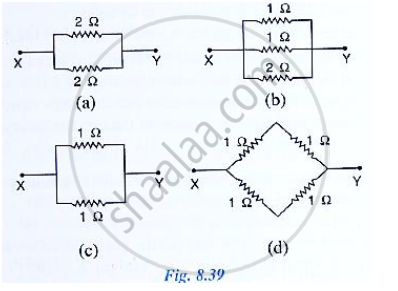#### Chapter 8: Current Electricity solutions [Page 0]

The diagram below in Fig. 8.40 shows a cell of e.m.f. ε = 2 volt and internal resistance r = 1 ohm to an external resistance R = 4 ohm. The ammeter A measures the current in the circuit and the
voltmeter V measures the terminal voltage across the cell. What will be the readings of the ammeter and voltmeter when (i) the key K is open, (ii) the key K is closed.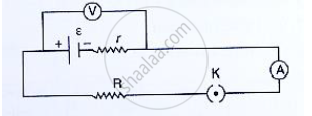A battery of e.m.f 3.0 V supplies current through a circuit in which the resistance can be changed.
A high resistance voltmeter is connected across the battery. When the current is 1.5 A, the voltmeter reads 2.7 V. Find the internal resistance of the battery.

A cell of e.m.f. 1.8V and internal resistance 2Ω is connected in series with an ammeter of resistance 0.7Ω and a resistor of 4.5Ω as shown in Fig. 8.41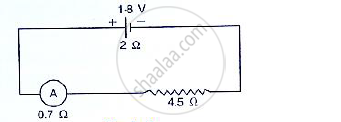(a) what would be the reading of the ammeter?
(b) what is the potential difference across the terminals of the cell?

A battery of e.m.f. 15 V and internal resistance 3 ohm is connected to two resistors of resistances 3 ohm and 6 ohm is series Find:
(a) the current through the battery
(b) the p.d. between the terminals of the battery.

A cell of e.m.f. ε and internal resistance 𝔯 sends current 1.0 A when it is connected to an external resistance 1.9Ω. But it sends current 0.5 A when it is connected to an external resistance 3.9 Ω.
Calculate the values of ε and 𝔯.

Two resistors having resistance 4𝛀 and 6𝛀 are connected in parallel. Find their equivalent resistance.

Four resistors each of resistance 2Ω are connected in parallel. What is the effective resistance?

You have three resistors of values 2Ω, 3Ω and 5Ω. How will you join them so that the total  resistance is less than 1Ω? Draw diagram and find the total resistance.

Three resistors each of 2Ω are connected together so that their total resistance is 3Ω. Draw a diagram to show this arrangement and check it by calculation.

Calculate the equivalent resistance of the following combination of resistors r1, r2, r3 and r4 if r_1=r_2=r_3=r_4=2.0Ω  between the points A and B in Fig. 8.42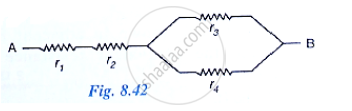A combination consists of three resistors in series. Four similar sets are connected in parallel. If the resistance of each resistor is 2 ohm, find the resistance of the combination.

In the circuit shown below in Fid 8.43, calculate the value of x if the equivalent resistance between A and B is 4Ω.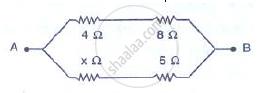Calculate the effective resistance between the points A and B in the circuit shown in Figure 8.44.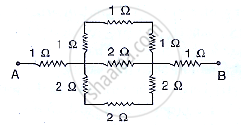A wire of uniform thickness with a resistance of 27Ω is cut into three equal pieces and they are joined in parallel. Find the equivalent resistance of the parallel combination.

A circuit consists of a 1 ohm resistor in series with a parallel arrangement of 6 ohm and 3 ohm resistors. Calculate the total resistance if the circuit. Draw a diagram.

Calculate the effective resistance between the points A and B in the network shown below in Figure 8.45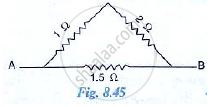Calculate the equivalent resistance between A and B in the adjacent diagram in Figure 8.46.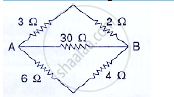In the network shown in adjacent Fig. 8.47, calculate the equivalent resistance between the points.

(a) A and B
(b) A and C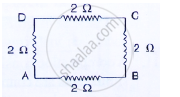Five resistors, each 3 Ω, are connected as shown in Fig 8.48. Calculate the resistance (a) between the points P and Q. (b) between the points X and Y.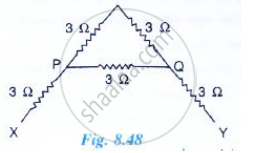Two resistors of 2.0Ω and 3.0Ω are connected (a) in series (b) in parallel, with a battery of 6.0 V and negligible internal resistance. For each case draw a circuit diagram and calculate the current through the battery.

A resistor of 6Ω is connected in series with another resistor of 4 Ω. A potential difference of 20 V is applied across the combination. Calculate (a) the current in the circuit and (b) the potential difference across the 6Ω resistor.

In figure 8.50, calculate:
(a) the total resistance of the circuit
(b) the value if R, and
(c) the current flowing in R.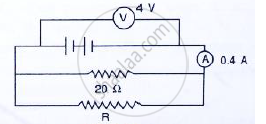A wire of length 5 m has a resistance of 2.0 Ω calculate:
(a) the resistance of wire of length 1 m
(b) the equivalent resistance if two such wires each of length 2 m are joined in parallel.
(c) the resistance of 1 m length of wire of same material but of half diameter.

A particular resistance wire has a resistance of 3.0 ohm per meter. Find:
(a) The total resistance of three lengths of this wire each 1.5 m long, joined in parallel.
(b) The potential difference of the battery which gives a current od 2.0 A in each of the 1.5 m length when connected in parallel to the battery (assume that the resistance of battery is negligible)
(c) the resistance of 5 m length of a wire of the same material, but with twice the area of cross
section.

A cell supplies a current of 1.2 A through two 2 Ω resistors connected in parallel. When the resistors are connected in series, it supplies a current of 0.4 A. Calculate: (i) the internal resistance and (ii) e.m.f. of the cell.

A battery of e.m.f 15 V and internal resistance 3 Ω is connected to two resistors 3Ω and 6Ω connected in parallel. Find: (a) the current through the battery. (b) p.d. between the terminals of the battery, (c) the current in 3 Ω resistors, (d) the current in 6 Ω resistor.

The following circuit diagram (Fig . 8.51) shows three resistors 2Ω, 4Ω and RΩ connected to a battery of e.m.f 2 V and internal resistance 3Ω. A main current of 0.25A flows through the circuit.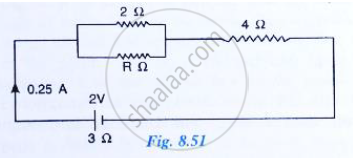Three resistors of 6.0 𝛀, 2.0𝛀 and 4.0𝛀 are joined to an ammeter A and a cell of e.m.f. 6.0 V as
shown in fig 8.52 Calculate:
(a) the effective resistance of the circuit and
(b) the reading of ammeter.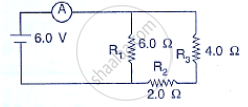The diagram below in Fig. 8.53 shows the arrangement of five different resistances connected
to a battery of e.m.f. 1.8V Calculate:
(a) the total resistance of the circuit, and
(b) the reading of ammeter A.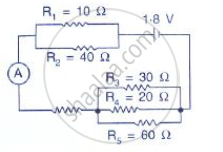## Chapter 8: Current Electricity

#### Selina Selina ICSE Concise Physics Class 10## Selina solutions for Class 10 Physics chapter 8 - Current Electricity

Selina solutions for Class 10 Physics chapter 8 (Current Electricity) include all questions with solution and detail explanation. This will clear students doubts about any question and improve application skills while preparing for board exams. The detailed, step-by-step solutions will help you understand the concepts better and clear your confusions, if any. Shaalaa.com has the CISCE Selina ICSE Concise Physics for Class 10 solutions in a manner that help students grasp basic concepts better and faster.

Further, we at Shaalaa.com are providing such solutions so that students can prepare for written exams. Selina textbook solutions can be a core help for self-study and acts as a perfect self-help guidance for students.

Concepts covered in Class 10 Physics chapter 8 Current Electricity are Concept of Current Electricity, Resistance of a System of Resistors - Resistors in Series, Internal Resistance, Concepts of Pd (V), Current (I), Resistance (R) and Charge (Q)., Electric Potential Difference, Resistance of a System of Resistors - Resistors in Parallel, Concepts of Emf, Ohm’s Law, Concept of Current Electricity Numericals.

Using Selina Class 10 solutions Current Electricity exercise by students are an easy way to prepare for the exams, as they involve solutions arranged chapter-wise also page wise. The questions involved in Selina Solutions are important questions that can be asked in the final exam. Maximum students of CISCE Class 10 prefer Selina Textbook Solutions to score more in exam.

Get the free view of chapter 8 Current Electricity Class 10 extra questions for Physics and can use Shaalaa.com to keep it handy for your exam preparation

S